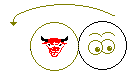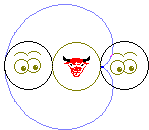# Two coins puzzle

Place two identical coins side by side and roll one along the circumference of another without slipping. (Does it remind you of the way to draw epicycloids?). Two very related questions may now be asked:

1. What will be the position of the rolling coin when it gets to the other side of the coin at rest, i.e., after traversing only one half of the circumference of the latter? Will it be upside down or the right side up?

2. How many revolutions will the rolling coin undergo before it returns to its original position?The puzzle is actually an old-timer but never fails to surprise people who never saw it before. Even with the solution suggested by the picture on the right, it takes some experimenting before finally but still with the feeling of disbelief the right answer is accepted:

1. The coin will be the right side up.

2. It will take two revolutions for the rolling coin to get back to its starting position.

The puzzle illustrates how shaky our intuition about motion (and, by extension, intuition in general) may be. From observing drawing of the epicycloid it becomes apparent that the point on the rolling coin nearest to the other coin (i.e. the point where the two coins touch) will be the farthest from the latter after the rolling coin traverses a half-circumference. It's not yet a proof but an experiment that might help our intuition to set things straight.

The ultimate proof comes from the following observation. The rolling coin actually participates in two separate motions not unlike the moon relative to the earth:

• It rotates around its own center.
• It rotates around the center of the other coin.To answer the first question, note that in both motions the moving coin makes a half-turn. A half-turn around a point can be described as a reflection in that point. Choose three points A,B,C on the rolling coin. When reflected in a point O, A is carried into A' while, B and C are carried into B' and C', respectively. Another reflection in a point O', moves A', B', C' into A", B", and C", respectively. Now note, that O is the middle point of the segments AA' and BB' whereas O' is the middle point of two segments A'A" and B'B". Therefore, in both triangles AA'A" and BB'B", the line OO' connects midpoints of two sides thus being parallel and equal to half size of the third side. From here, AA" and BB" are equal and parallel (both, of course, are equal and parallel to CC" as well.) Since points A,B,C have been chosen arbitrary on the moving coin, we can now say that the whole coin was translated along a vector AA".